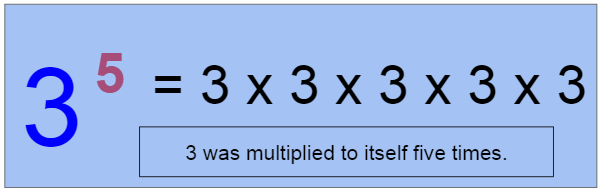# Laws Of Indices

Contents

## LEARNING OBJECTIVES:

• To define index and base of a number
• To discuss the different laws of indices
• To evaluate expressions using the laws of indices

## MATH CONCEPTS

• INDEX OF A NUMBER- The index of a number tells how many times to use the number in a multiplication. It is written as a small number to the right and above the base number. It is also known as exponent or power.
• BASE OF A NUMBER- It is the number that gets multiplied when using an index or exponent.
• THE THREE BASIC LAWS OF INDICES For any real number x, y, m, and n, the following rules uphold:
• Multiplication Law/ Product Rule$x^{m}\cdot x^{n}=x^{m+n}$
• Division Law/Quotient Rule$x^{m}/x^{n}=\frac{x^{m}}{x^{n}}=x^{m-n}$
• Power Law/Power Rule$(x^{m})^{n}=x^{m\cdot n}$
• Other Laws:
•$x^{-n} = \frac{1}{x^{n}}$
•$x^{\frac{m}{n}}=\sqrt[n]{x^{m}}$
•$(xy)^{m}=x^{m}y^{m}$
•$(\frac{x}{y})^{n}=\frac{x^{n}}{y^{n}}$
•$x^{0}=1,\,x\neq 0$

IMPORTANCE

• Understanding the laws of indices plays an important role in manipulating and simplifying expressions involving indices.
• Laws of indices can also be used to evaluate expressions involving indices without the use of calculators.

## DISCUSSION

BASE AND INDEX OF A NUMBERIt is vital to get familiarized with the base and index of a number. This will become the foundation of learning the laws of indices. In the given example, 3 is raised to 5. Meaning, the base will be multiplied to itself five times.If we will evaluate the given, 3 x 3 x 3 x 3 x 3 = 243.

## DISCUSSION

LAWS OF INDICES

• Multiplication Law/ Product RuleWhen expressions with the same base (x) are multiplied, the indices (m and n) are added.$x^{m}\cdot x^{n}=x^{m+n}$

Examples:

1)$2^{a}\cdot 2^{b}=2^{a+b}$        2)$4^{\frac{1}{2}}\cdot 4^{\frac{1}{3}}=4^{\frac{1}{6}}$        3)$z^{5}\cdot z^{4}=z^{9}$

• Division Law/ Quotient RuleWhen expressions with the same base (x) are divided, the indices (m and n) are subtracted.$x^{m}/x^{n}=\frac{x^{m}}{x^{n}}=x^{m-n}$

Examples:

1)$5^{3a}/5^{2b}=5^{3a-2b}$                2)$10^{6}/10^{2}=10^{6-2}=10^{4}$        3)$y^{2a}/y^{4}=y^{2a-4}$

• Power Law/ Power RuleWhen an expression is raised to a certain index and is raised again to another index, the indices (m and n) are multiplied.$(x^{m})^{n}=x^{m\cdot n}$

Examples:

1)$(6^{5p})^{2q}=6^{5p\cdot 2q}$                 2)$(9^{8})^{2}=9^{8\cdot 2}=9^{16}$                 3)$(c^{4})^{n}=c^{4\cdot n}=c^{4n}$

ILLUSTRATIVE EXAMPLES

Find the value of the following series.

1.$(c^{2}\cdot c^{8})/c^{5}=c^{10}/c^{5}=c^{10-5}=c^{5}$
2.$\frac{6^{13}}{6^{2}\cdot 6^{3}}=\frac{6^{13}}{6^{2(3)}}=\frac{6^{13}}{6^{6}}=6^{13-6}=6^{7}$
3.$m^{-2}n^{3}\cdot m^{5}n^{-4}=m^{-2+5}n^{3-4}=m^{3}n^{-1}=\frac{m^{3}}{n}$
4.$(p^{3}q^{5})^{3}=(p^{3(3)}q^{5(3)})=p^{9}q^{15}$
5.$3(4x^{4}y^{3})^{2}=3(4x^{4(2)}y^{3(2)})=3(4x^{8}y^{6})=12x^{8}3y^{6}$
6.$\frac{rs^{2}t^{3}}{s^{-5}t^{2}}=rs^{2+5}t^{3-2}=rs^{7}t$
7.$\sqrt{\frac{b^{4}}{c^{-2}}}=b^{\frac{4}{7}}c^{\frac{2}{7}}$
8.$\frac{a^{2}b^{\frac{1}{3}}}{a^{\frac{1}{2}}b^{3}}=a^{2-\frac{1}{2}}b^{\frac{1}{3}-3}=\frac{a\frac{3}{2}}{b^{\frac{8}{3}}}$

REFERENCES: